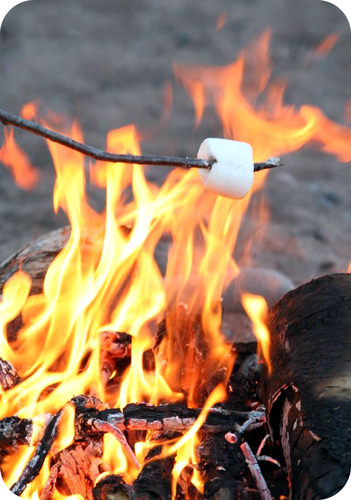# 11.6: Combustion Reactions

$$\newcommand{\vecs}{\overset { \rightharpoonup} {\mathbf{#1}} }$$ $$\newcommand{\vecd}{\overset{-\!-\!\rightharpoonup}{\vphantom{a}\smash {#1}}}$$$$\newcommand{\id}{\mathrm{id}}$$ $$\newcommand{\Span}{\mathrm{span}}$$ $$\newcommand{\kernel}{\mathrm{null}\,}$$ $$\newcommand{\range}{\mathrm{range}\,}$$ $$\newcommand{\RealPart}{\mathrm{Re}}$$ $$\newcommand{\ImaginaryPart}{\mathrm{Im}}$$ $$\newcommand{\Argument}{\mathrm{Arg}}$$ $$\newcommand{\norm}{\| #1 \|}$$ $$\newcommand{\inner}{\langle #1, #2 \rangle}$$ $$\newcommand{\Span}{\mathrm{span}}$$ $$\newcommand{\id}{\mathrm{id}}$$ $$\newcommand{\Span}{\mathrm{span}}$$ $$\newcommand{\kernel}{\mathrm{null}\,}$$ $$\newcommand{\range}{\mathrm{range}\,}$$ $$\newcommand{\RealPart}{\mathrm{Re}}$$ $$\newcommand{\ImaginaryPart}{\mathrm{Im}}$$ $$\newcommand{\Argument}{\mathrm{Arg}}$$ $$\newcommand{\norm}{\| #1 \|}$$ $$\newcommand{\inner}{\langle #1, #2 \rangle}$$ $$\newcommand{\Span}{\mathrm{span}}$$$$\newcommand{\AA}{\unicode[.8,0]{x212B}}$$Figure $$\PageIndex{1}$$ (Credit: Nina Hale; Source: http://commons.wikimedia.org/wiki/File:RoastingMarshmallow.jpg(opens in new window); License: CC by 2.0(opens in new window))

### How do you cook the perfect marshmallow?

Roasting marshmallows over an open fire is a favorite past-time for campers, outdoor cook-outs, and just gathering around a fire in the back yard. The trick is to get the marshmallow a nice golden brown without catching it on fire. Too often we are not successful and we see the marshmallow burning on the stick – a combustion reaction taking place right in front of us.

## Combustion Reactions

A combustion reaction is a reaction in which a substance reacts with oxygen gas, releasing energy in the form of light and heat. Combustion reactions must involve $$\ce{O_2}$$ as one reactant. The combustion of hydrogen gas produces water vapor:

$2 \ce{H_2} \left( g \right) + \ce{O_2} \left( g \right) \rightarrow 2 \ce{H_2O} \left( g \right)\nonumber$

Notice that this reaction also qualifies as a combination reaction.Figure $$\PageIndex{2}$$: Explosion of the Hindenberg. (Credit: Courtesy of Gus Pasquerella/US Navy; Source: http://commons.wikimedia.org/wiki/File:Hindenburg_burning.jpg(opens in new window); License: Public Domain)

The Hindenberg was a hydrogen-filled airship that suffered an accident upon its attempted landing in New Jersey in 1937. The hydrogen immediately combusted in a huge fireball, destroying the airship and killing 36 people. The chemical reaction was a simple one: hydrogen combining with oxygen to produce water.

Many combustion reactions occur with a hydrocarbon, a compound made up solely of carbon and hydrogen. The products of the combustion of hydrocarbons are carbon dioxide and water. Many hydrocarbons are used as fuel because their combustion releases very large amounts of heat energy. Propane $$\left( \ce{C_3H_8} \right)$$ is a gaseous hydrocarbon that is commonly used as the fuel source in gas grills.

$\ce{C_3H_8} \left( g \right) + 5 \ce{O_2} \left( g \right) \rightarrow 3 \ce{CO_2} \left( g \right) + 4 \ce{H_2O} \left( g \right)\nonumber$

##### Example $$\PageIndex{1}$$: Combustion Reactions

Ethanol can be used as a fuel source in an alcohol lamp. The formula for ethanol is $$\ce{C_2H_5OH}$$. Write the balanced equation for the combustion of ethanol.

###### Solution

Step 1: Plan the problem.

Ethanol and oxygen are the reactants. As with a hydrocarbon, the products of the combustion of an alcohol are carbon dioxide and water.

Step 2: Solve.

Write the skeleton equation:

$\ce{C_2H_5OH} \left( l \right) + \ce{O_2} \left( g \right) \rightarrow \ce{CO_2} \left( g \right) + \ce{H_2O} \left( g \right)\nonumber$

Balance the equation.

$\ce{C_2H_5OH} \left( l \right) + 3 \ce{O_2} \left( g \right) \rightarrow 2 \ce{CO_2} \left( g \right) + 3 \ce{H_2O} \left( g \right)\nonumber$

Combustion reactions must have oxygen as a reactant. Note that the water produced is in the gas state, rather than the liquid state, because of the high temperatures that accompany a combustion reaction.

## Summary

• Combustion reaction is defined and examples are given.

## Review

1. What is needed for a combustion reaction to take place?
2. What is formed in any combustion reaction?
3. Mercury reacts with oxygen to form mercuric oxide. Is this a combustion reaction?
4. What are the products of any combustion reaction involving a hydrocarbon?

This page titled 11.6: Combustion Reactions is shared under a CK-12 license and was authored, remixed, and/or curated by CK-12 Foundation via source content that was edited to the style and standards of the LibreTexts platform; a detailed edit history is available upon request.